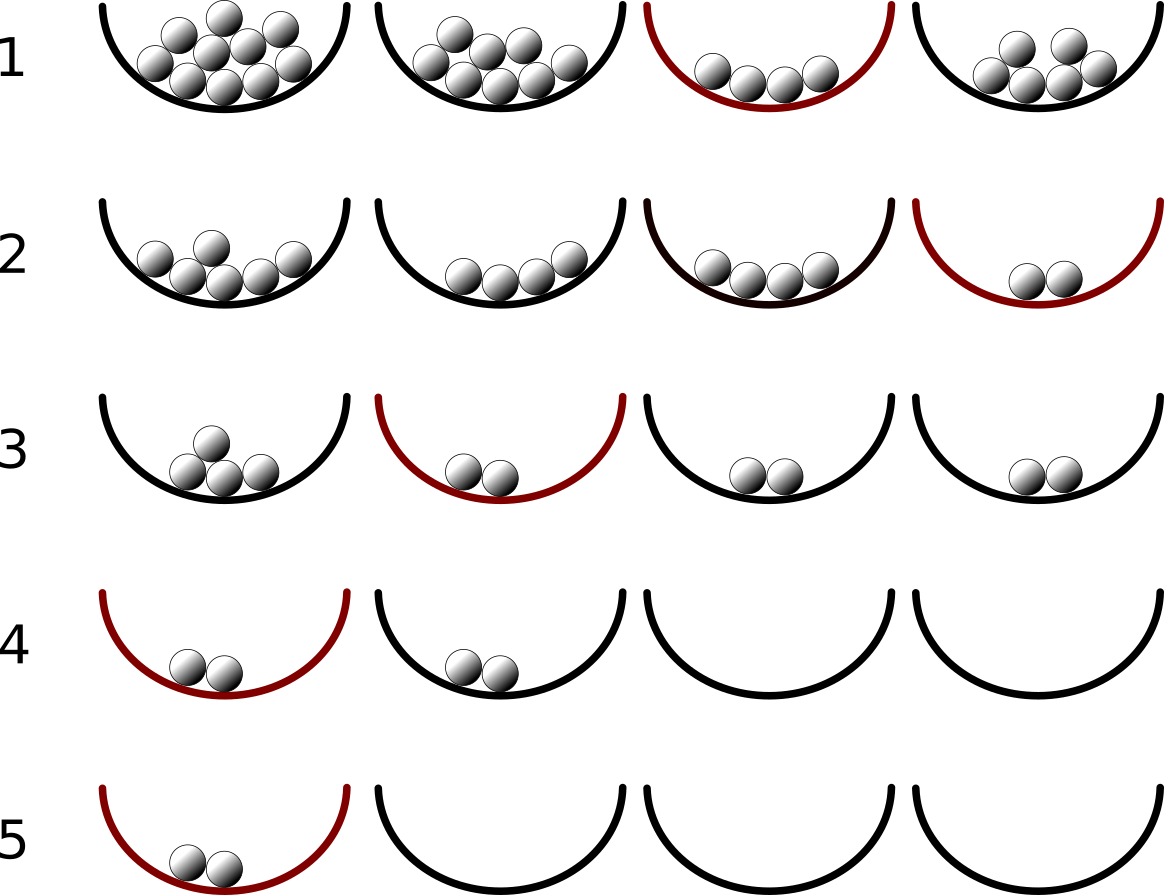시간 제한메모리 제한제출정답맞힌 사람정답 비율
2 초 1024 MB16151292.308%

## 문제You can find your lucky number of the day in the following way.

Prepare four bowls and plenty of marbles. Line up the bowls from left to right, and, after deep meditation, put some arbitrary numbers of marbles in the bowls. Numbers of marbles in the bowls may vary; some bowls may be empty, but at least one of them should be non-empty.

Among non-empty bowls, choose one with the least number of marbles in it. If more than one bowl has the least number of marbles, choose the leftmost. From all the other non-empty bowls, remove the same number of marbles as in the chosen bowl. The marbles in the chosen bowl are left there.

This procedure should be repeated until only one non-empty bowl remains. The number of marbles in the non-empty bowl is your lucky number today.The figure to the right shows an example corresponding to the first dataset of the Sample Input.

1. The bowl second from the right contains the least number of marbles, four.
2. Four marbles each have been taken out from other bowls. Now the rightmost bowl has two marbles, which are the least.
3. Two marbles each have been taken out from other bowls. Three bowls except the leftmost have two marbles, which are the least. The leftmost bowl of the three, that is, the second from the left, is chosen.
4. Two marbles each have been taken out from other bowls. Now only two bowls have marbles in them and both have two marbles. Thus, the leftmost bowl is chosen.
5. Two marbles from the second bowl have been taken out, leaving only one bowl non-empty, which is the leftmost bowl with two marbles. Yes, your lucky number of the day is two!

## 입력

The input consists of multiple datasets, each in the following format.

a1 a2 a3 a4

Each of the datasets consists of one line containing four integers, a1 through a4. These four integers are the numbers of marbles initially in the four bowls. All of these four integers are between 0 and 100, inclusive, and at least one of them is non-zero.

The end of the input is indicated by a line containing four zeros.

The number of datasets does not exceed 100.

## 출력

For each of the datasets, output the lucky number decided by the procedure described above in a line.

## 예제 입력 1

10 8 4 6
0 21 7 35
5 45 13 3
52 13 91 78
0 0 0 0


## 예제 출력 1

2
7
1
13